Students can Download Tamil Nadu 12th Physics Model Question Paper 2 English Medium Pdf, Tamil Nadu 12th Physics Model Question Papers helps you to revise the complete Tamilnadu State Board New Syllabus, helps students complete homework assignments and to score high marks in board exams.

## TN State Board 12th Physics Model Question Paper 2 English Medium

General Instructions:

• The question paper comprises of four parts.
• You are to attempt all the parts. An internal choice of questions is provided wherever applicable.
• All questions of Part I, II, III, and IV are to be attempted separately.
• Question numbers 1 to 15 in Part I are Multiple Choice Questions of one mark each.
These are to be answered by choosing the most suitable answer from the given four
alternatives and writing the option code and the corresponding answer
• Question numbers 16 to 24 in Part II are two-mark questions. These are to be answered
in about one or two sentences.
• Question numbers 25 to 33 in Part III are three-mark questions. These are to be answered
in about three to five short sentences.
• Question numbers 34 to 38 in Part IV are five-mark questions. These are to be answered
in detaiL Draw diagrams wherever necessary.

Time: 3 Hours
Max Marks: 70

Part – I

Answer all the questions. Choose the correct answer. [15 x 1 = 15]

Question 1.
Three capacitors are connected in triangle as shown in the figure. The equivalent capacitance between points A and C is……………………………..
(a) 1 μF
(b) 2 μF
(c) 3 μF
(d) 1/4 μF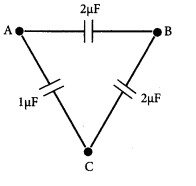(b) 2 μF

Question 2.
If the electric field in a region is given by $$\overrightarrow{\mathrm{E}}=5 \hat{i}+4 \hat{j}+9 \hat{k}$$, then the electric flux through a surface of area 20 units lying in the
y – z plane will be …………………
(a) 20 units
(b) 80 units
(c) 100 units
(d) 180 units
(c) 100 units
Hint. The area vector
$$\overrightarrow{\mathrm{A}}=20 \hat{i} ; \overrightarrow{\mathrm{E}}=(5 \hat{i}+4 \hat{j}+9 \hat{k})$$
Flux ( φ)$$\overrightarrow{\mathrm{E}} \cdot \overrightarrow{\mathrm{A}}$$ = 5 x 20 = 100 unitsQuestion 3.
A wire of resistance 2 ohms per meter is bent to form a circle of radius 1 m. The equivalent resistance between its two diametrically opposite points, A and B as shown in the figure is(a) π Ω
(b) $$\frac{\pi}{2} \Omega$$
(c) 2π Ω
(d) $$\frac{\pi}{4} \Omega$$
(b) $$\frac{\pi}{2} \Omega$$

Question 4.
A non-conducting charged ring of charge q, mass m and radius r is rotated with constant angular speed co. Find the ratio of its magnetic moment with angular momentum is …………..
(a) M
(b) $$\frac{3}{\pi} \mathrm{M}$$
(c) $$\frac{2}{\pi} \mathrm{M}$$
(d) $$\frac{1}{2} \mathrm{M}$$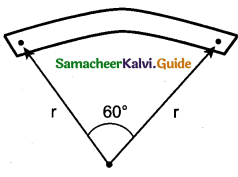(b) $$\frac{3}{\pi} \mathrm{M}$$

Question 5.
A proton enters a magnetic field of flux density 1.5 Wb/m2 with a speed of 2 x 107 m/s at angle of 30° with the field. The force on the proton will be ……………….
(a) 0.24 x 10-12 N
(b) 2.4 x 10 -12 N
(c) 24 x 10-12 N
(d) 0.024 x 10-12 N
(b) 2.4 x 10 -12 N
Hint: F = Bqv sin θ = 1.5 x 1.6 x 10-19 x 2 x 107 x sin 30°= 2.4 x 10-12 N

Question 6.
In an electrical circuit, R, L, C and AC voltage source are all connected in series. When L is removed from the circuit, the phase difference between the voltage and current in the circuit is $$\frac{\pi}{3}$$ Instead, if C is removed from the circuit, the phase difference is again $$\frac{\pi}{3}$$ . The power factor of the of the circuit is ……………
(a) $$\frac{1}{2}$$
(b) $$\frac{1}{\sqrt{2}}$$
(c) 1
(d) $$\frac{\sqrt{3}}{2}$$
(c) 1Question 7.
The inductance of a coil is proportional to…………………………………….
(a) its length
(b) the number of turns
(c) the resistance of the coil
(d) square of the number of turns
(d) square of the number of turns

Question 8.
The electric and magnetic fields of an electromagnetic wave are……………….
(a) in phase and perpendicular to each other
(b) out of phase and not perpendicular to each other
(c) in phase and not perpendicular to each other
(d) out of phase and perpendicular to each other
(a) in phase and perpendicular to each other

Question 9.
One of the of Young’s double slits is covered with a glass plate as shown in figure. The position of central maximum will ……………
(a) get shifted downwards
(b) get shifted upward
(c) will remain the same
(d) data insufficient to conclude(b) get shifted upward

Question 10.
The wavelength λe of an electron and λp of a photon of same energy E are related ………..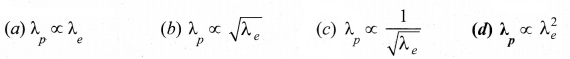(d) $$\lambda_{p} \propto \lambda_{e}^{2}$$Question 11.
A system consists of No nucleus at t = 0. The number of nuclei remaining after half of a half-life (that  is, at time $$t=\frac{1}{2} \mathrm{T}_{\frac{1}{2}}$$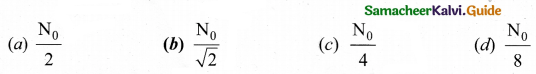Hint: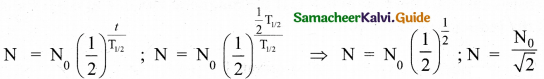Question 12.
In a pure semiconductor crystal, if current flows due to breakage of crystal bonds, then the semiconductor is called……………………………….
(a) acceptor
(b) donor
(c) intrinsic semiconductor
(d) extrinsic semiconductor
(c) intrinsic semiconductor
Hint: Pure semiconductors are called intrinsic semiconductors.Question 13.
The light emitted in an LED is due to……………………………
(a) Recombination of charge carriers
(b) Reflection of light due to lens action
(c) Amplification of light falling at the junction
(a) Recombination of charge carriers

Question 14.
The frequency range of 3 MHz to 30 MHz is used for………………………………..
(a) Ground wave propagation
(b) Space wave propagation
(c) Sky wave propagation
(d) Satellite communication
(c) Sky wave propagation

Question 15.
The materials used in Robotics are……………………..
(a) Aluminium and silver
(b) Silver and gold
(c) Copper and gold
(d) Steel and aluminium
(d) Steel and aluminium

Part – III

Answer any six questions. Question No. 20 is compulsory.   [6×2 = 12]

Question 16.
Define ‘Electric dipole’
Two equal and opposite charges separated by a small distance constitute an electric dipole.Question 17.
Define current density.
The current density (J) is defined as the current per unit area of cross section of the conductor
$$\mathrm{J}=\frac{\mathrm{I}}{\mathrm{A}}$$
The S.I. unit of current density. $$\frac{\mathrm{A}}{\mathrm{m}^{2}}(\text { or }) \mathrm{Am}^{-2}$$

Question 18.
What is magnetic susceptibility?
It is defined as the ratio of the intensity of magnetisation $$(\overrightarrow{\mathrm{M}})$$ induced in the material due to magnetising field $$(\overrightarrow{\mathrm{H}})$$
$$\chi_{m}=\left|\frac{\overrightarrow{\mathrm{M}}}{\overrightarrow{\mathrm{H}}}\right|$$

Question 19.
What is meant by electromagnetic induction?
Whenever the magnetic flux linked with a closed coil changes, an emf (electromotive force) is induced and hence an electric current flows in the circuit.

Question 20.
A coil of 200 turns carries a current of 0.4 A. If the magnetic flux of 4 mWb is linked with the coil, find the inductance of the coil.
Number of turns, N = 200; Current, I = 0.4 A
Magnetic flux linked with coil, φ = 4 mWb = 4 x 10-3 Wb
Induction of the coil , L
$$\mathrm{L}=\frac{\mathrm{N} \phi}{\mathrm{I}}=\frac{200 \times 4 \times 10^{-3}}{0.4}=\frac{800 \times 10^{-3}}{0.4}=2 \mathrm{H}$$Question 21.
Why do stars twinkle?
The stars actually do not twinkle. They appear twinkling because of the movement of the atmospheric layers with varying refractive indices which is clearly seen in the night sky.

Question 22.
How many photons of frequency 1014 Hz will make up 19.86 J of energy?
Total energy emitted per second = Power x time
19.863 = Power x is
∴ Power 19.86 W
Number of photons =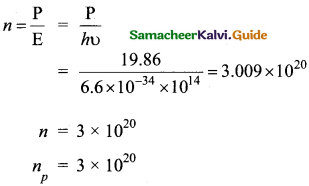Question 23.
Define curie.
One curie was defined as number of decays per second in 1 g of radium and it is equal to 3.7 x 1010 decays/s

Question 24.
A transistor having α =0.99 and VBE = 0.7V, is given in the circuit. Find the value of the collector current.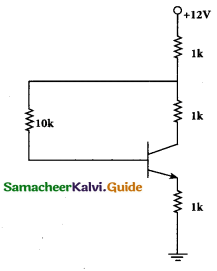Part – III

Answer any six questions. Question No. 26 is compulsory.   [6 x 3 = 18]

Question 25.
Write a short note on ‘electrostatic shielding’.
Consider a cavity inside the conductor. Whatever the charges at the surfaces and whatever the electrical disturbances outside, the electric field inside the cavity is zero. A sensitive electrical instrument which is to be protected from external electrical disturbance is kept inside this cavity. This is called electrostatic shielding.Question 26.
A conductor of linear mass density 0.2 g m-1 suspended ,by two flexible wire as shown in figure. Suppose the tension in the supporting wires is zero when it is kept inside the magnetic field of 1 T whose direction is into the x page. Compute the current inside the conductor and also the direction of the current. Assume g = 10 m s-2 = 111.87.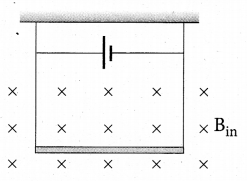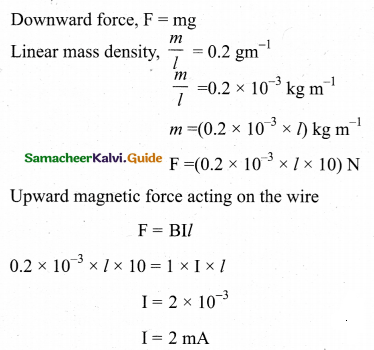Question 27.
How is Eddy current produced? How do they flow in a conductor?
Even for a conductor in the form of a sheet or plate, an emf is induced when magnetic flux linked with it changes. But the difference is that there is no definite loop or path for induced current to flow away. As a result, the induced currents flow in concentric circular paths. As these electric currents resemble eddies of water, these are known as Eddy currents. They are also called Foucault currents.

Question 28.
Explain the concept of intensity of electromagnetic waves.
The energy crossing per unit area per unit time and perpendicular to the direction of propagation of electromagnetic wave is called the intensity.
Intensity, I = (u)c

Question 29.
If the focal length is 150 cm for a glass lens, what is the power of the lens?
Given: focal length,f = 150 cm (or) f= 1.5 m
Equation for power of lens is, P = 1/f
Substituting the values,
$$P = \frac{1}{1.5}$$= 0.067 diopter
As the power is positive, it is a converging lens.Question 30.
A proton and an electron have same kinetic energy. Which one has greater de Broglie wavelength. Justify.
de-Broglic wavelength of the particle is $$\lambda=\frac{h}{p}=\frac{h}{\sqrt{2 m \mathrm{K}}}$$ $$\text { i.e. } \lambda \propto \frac{1}{\sqrt{m}}$$
As me <<mp ,so λe >>λP
Hence protons have greater de- broglic wavelength

Question 31.
Distinguish between avalanche and zener breakdown.

 Avalanche Breakdown Zener Breakdown It occurs injunctions which are lightly and have wide depletion widths. It occurs in junctions which are heavily doped and have narrow depletion widths. It occurs at higher reverse voltages when thermally generated electrons get enough kinetic energy to produce more electrons by collision. It occurs due to rupture of covalent bonds by strong electric fields set up in depletion region by the reverse voltage. At reverse voltage above 6V breakdown is due to avalanche effect. At reverse voltage below 6V breakdown is due to zener effect. Electric field produced is weak in nature. A strong electric field is produced Charge carriers obtain energy from the applied potential. Zener current is independent of applied voltage.

Question 32.
Explain centre frequency or resting frequency in frequency modulation.
When the frequency of the baseband signal is zero (no input signal), there is no change in the frequency of the carrier wave. It is at its normal frequency and is called as centre frequency or resting frequency.Question 33.
What are black holes?
Black holes are end stage of stars Which are highly dense massive object. Its mass ranges from 20 times mass of the sun to 1 million times mass of the sun. It has very strong gravitational force such that no particle or even light can escape from it. The existence of black holes is studied when the stars orbiting the black hole behave differently from the other starts. Every galaxy has black hole at its center. Sagittarius A* is the black hole at the center of the Milky Way galaxy.

Part – IV

Answer all the questions.  [5 x 5 = 25]

Question 34.
(a) How do we determine the electric field due to a continuous charge distribution? Explain. Electric field due to continuous charge distribution
The electric charge is quantized microscopically. The expressions of Coulomb’s Law, superposition principle force and electric field are applicable to only point charges. While dealing with the electric field due to a charged sphere or a charged wire etc., it is very difficult to look at individual charges in these charged bodies.Therefore, it is assumed that charge is distributed continuously on the charged bodies and the discrete nature of charges is not considered here. The electric field due to such continuous charge distributions is found by invoking the method of calculus.

Consider the following charged object of irregular shape. The entire charged object is divided into a large number of charge elements
Δq1, Δq2, Δq3 ….. Δqn ……… and each charge element Δq is taken as a point charge.
The electric field at a point P due to a charged object is approximately given by the sum of the fields at P due to all such change elementsHere Δ qi is the ith charge element, r is the distance of the point P from the zth charge element and $$\hat{r}_{i \mathrm{P}}$$ is the unit vector from ith charge element to the point P.

However, the equation is only an approximation. To incorporate the continuous distribution of charge, we take the limit Δq → 0(= dq). In this limit, the summation in the equation becomes an integration and takes the following form
$$\overrightarrow{\mathrm{E}}=\frac{1}{4 \pi \varepsilon_{0}} \int \frac{d q}{r^{2}} \hat{r}$$

Here r is the distance of the point P from the infinitesimal charge dq and $$\hat{r}$$ is the unit vector from dq to point P. Even though the electric field for a continuous charge distribution is difficult to evaluate, the force experienced by some test charge q in this electric field is still given by $$\overrightarrow{\mathrm{F}}=q \overrightarrow{\mathrm{E}}$$

(a) Line charge distribution: If the charge Q is uniformly distributed along the wire of length L, then linear charge density (charge per unit length) is λ = Q/L. Its unit is coulomb per meter (Cm-1). The charge present in the infinitesimal length dl is dq = λ dl
The electric field due to the line of total charge Q is given by(b) Surface charge distribution: If the charge Q is uniformly distributed on. a surface of area A, then surface charge density (charge per unit area) is $$\lambda=\frac{Q}{L}$$. Its unit is coulomb per square meter (Cm-2 ). The charge present in the infinitesimal area dA is dq = adA. The electric field due to a of total charge Q is given by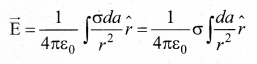(c) Volume charge distribution: If the charge Q is uniformly distributed in a volume V, then volume charge density (charge per unit volume) is given by $$\rho=\frac{Q}{V}$$ . Its unit is coulomb per cubic meter (Cm-3 ). The charge present in the infinitesimal volume element dV is dq = ρdV.
The electric field due to a volume of total charge Q is given by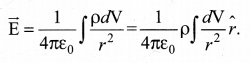[OR]

(b) Obtain the macroscopic form of Ohm’s law from its microscopic form and discuss its limitation.
Ohm’s law: The Ohm’s law can be derived from the equation J = σE. Consider a segment of wire of length l and cross sectional area A.When a potential difference V is applied across the wire, a net electric field is created in the wire which constitutes the current. For simplicity, we assume that the electric field is uniform in the entire length of the wire, the potential difference (voltage V) can be written as
V = El
As we know, the magnitude of current density
$$\mathrm{J}=\sigma \mathrm{E}=\sigma \frac{\mathrm{V}}{l}$$
But $$\mathrm{J}=\frac{\mathrm{I}}{\mathrm{A}}$$,so we write the equation as
$$\frac{\mathrm{I}}{\mathrm{A}}=\sigma \frac{\mathrm{V}}{l}$$
By rearranging the above equations, we get
$$\mathrm{V}=\mathrm{I}\left(\frac{l}{\sigma \mathrm{A}}\right)$$The quantity $$\frac{l}{\sigma \mathrm{A}}$$ is called resistance of the conductor and it is denoted as R. Note that the resistance is directly proportional to the length of the conductor and inversely proportional to area of cross section.
Therefore, the macroscopic form of Ohm’s law can be stated as
V = IR …………….. (3)

Question 35.
(a) Calculate the magnetic held inside and outside of the long solenoid using Ampere’s circuital law.
Magnetic field due to a long current carrying solenoid: Consider a solenoid of length L having N turns. The diametre of the solenoid is assumed to be much smaller when compared to its length and the coil is wound very closely.In order to calculate the magnetic field at any point inside the solenoid, we use Ampere’s circuital law. Consider a rectangular loop abed. Then from Ampere’s circuital law.
$$\oint_{C} \overrightarrow{\mathrm{B}} \cdot d \vec{l}=\mu_{0} \mathrm{I}_{\text {enclosed }}$$ = μ x (total current enclosed by Amperian loop)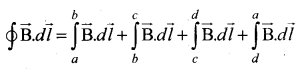Since the elemental lengths along be and da are perpendicular to the magnetic field which is along the axis of the solenoid, the integrals.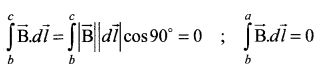Since the magnetic field outside the   solenoid is zero, the integral
$$\int_{c}^{d} \overrightarrow{\mathrm{B}} \cdot d \vec{l}=0$$
For the path along ab, the integral is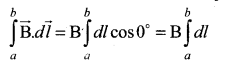where the length of the loop ab is h. But the choice of length of the loop ab is arbitrary. We can take very large loop such that it is equal to the length of the solenoid L. Therefore the integral is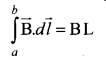Let NI be the current passing through the solenoid of N turns, thenThe number of turns per unit length is given by $$\frac{\mathrm{NI}}{\mathrm{L}}=n$$ then
$$\mathrm{v}_{p}=\varepsilon_{p}=-\mathrm{N}_{p} \frac{d \Phi_{\mathrm{B}}}{d t}$$
Since n is a constant for a given solenoid and μo is also constant. For a fixed current I, the magnetic field inside the solenoid is also a constant.

[OR]

(b) Explain the construction and working of transformer.
Construction and working of transformer:
Principle: The principle of transformer is the mutual induction between two coils. That is, when an electric current passing through a coil changes with time, an emf is induced in the neighbouring coil.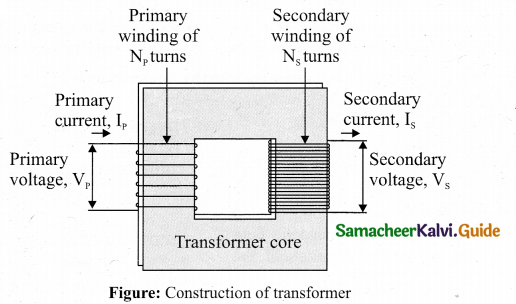Construction: In the simple construction of transformers, there are two coils of high mutual inductance wound over the same transformer core. The core is generally laminated and is made up of a good magnetic material like silicon steel. Coils are electrically insulated but magnetically linked via transformer core.

The coil across which alternating voltage is applied is called primary coil P and the coil from which output power is drawn out is called secondary coil S. The assembled core and coils are kept in a container which is filled with suitable medium for better insulation and cooling purpose.

Working: If the primary coil is connected to a source of alternating voltage, an alternating magnetic flux is set up in the laminated core. If there is no magnetic flux leakage, then whole of magnetic flux linked with primary coil is also linked with secondary coil. This means that rate at which magnetic flux changes through each turn is same for both primary and secondary coils.

As a result of flux change, emf is induced in both primary and secondary coils. The emf induced in the primary coil εp is almost equal and opposite to the applied voltage υp and is given by
$$\mathrm{v}_{p}=\varepsilon_{p}=-\mathrm{N}_{p} \frac{d \Phi_{\mathrm{B}}}{d t}$$
The frequency of alternating magnetic flux in the core is same as the frequency of the applied voltage. Therefore, induced emf in secondary will also have same frequency as that of applied voltage. The emf induced in the secondary coil εs is given by
$$\varepsilon_{\mathrm{s}}=-N_{s} \frac{d \Phi_{\mathrm{B}}}{d t}$$ ………………… (1)
where Np and Ns are the number of turns in the primary and secondary coil, respectively. If the secondary circuit is open, then  εs = υs where υs is the voltage across secondary coil.
$$v_{s}=\varepsilon_{s}=-\mathrm{N}_{s} \frac{d \Phi_{\mathrm{B}}}{d t}$$ ……….. (2)From equations (1) and (2),
$$\frac{v_{s}}{v_{p}}=\frac{N_{s}}{N_{p}}=K$$ …………………… (3)

This constant K is known as voltage transformation ratio. For an ideal transformer,
Input power υp ip= Output power υsis
where ipand is are the currents in the primary and secondary coil respectively. Therefore,
$$\frac{v_{s}}{v_{p}}=\frac{N_{s}}{N_{p}}=\frac{i_{p}}{i_{s}}$$ ………………….. (4)

Equation (4) is written in terms of amplitude of corresponding quantities,
$$\frac{V_{s}}{V_{p}}=\frac{N_{s}}{N_{p}}=\frac{I_{p}}{I_{s}}=K$$

(i) If Ns> Np ( or K > 1), ∴ Vs > Vp and Is < Ip. This is the case of step-up transformer in which voltage is increased and the corresponding current is decreased.

(ii) If Ns < Np (or K < 1) , ∴ Vs < Vp and Is > Ip . This is step-down transformer where voltage is decreased and the current is increased.

Question 36.
(a) Discuss the source of electromagnetic waves.
Sources of electromagnetic waves: Any stationary source charge produces only electric field. When the charge moves with uniform velocity, it produces steady current which gives rise to magnetic field (not time dependent, only space dependent) around the conductor in which charge flows. If the charged -particle accelerates, in addition to electric field it also produces magnetic field. Both electric and magnetic fields are time varying fields. Since the electromagnetic waves are transverse waves, the direction of propagation of electromagnetic waves is perpendicular to the plane containing electric and magnetic field vectors.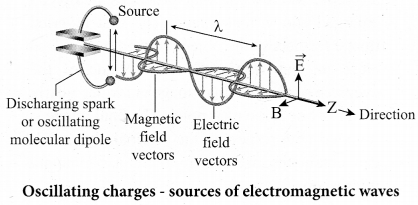Any oscillatory motion is also an accelerating motion, so, when the charge oscillates (oscillating molecular dipole) about their mean position, it produces electromagnetic waves. Suppose the electromagnetic field in free space propagates along z direction, and if the electric field vector points along y axis then the magnetic field vector will be mutually perpendicular to both electric field and the propagation vector direction, which means
Ey =E0 sin (kz-ωt)
Br = B0 sin(kz – ωt) where, Eo and Bo are amplitude of oscillating electric and magnetic field,$$\hat{k}$$ is a wave number, ω is the angular frequency of the wave and k (unit vector, here it is called propagation vector) denotes the direction of propagation of electromagnetic wave.

Note that both electric field and magnetic field oscillate with a frequency (frequency of electromagnetic wave) which is equal to the frequency of the source (here, oscillating charge is the source for the production of electromagnetic waves). In free space or in vacuum, the ratio between Eo and Bo is equal to the speed of electromagnetic wave, which is equal to speed of light c.
$$c=\frac{E_{0}}{B_{0}}$$

In any medium, the ratio of Eo and Bo is equal to the speed of electromagnetic wave in that medium, mathematically, it can be written as
$$v=\frac{E_{0}}{B_{0}}<c$$
Further, the energy of electromagnetic waves comes from the energy of the oscillating charge.[OR]

(b) Explain about compound microscope and obtain the equation for magnification.
Compound microscope:
The lens near the object, called the objective, forms a real, inverted, magnified image of the object. This serves as the object for the second lens which is the eyepiece. Eyepiece serves as a simple microscope that produces finally an enlarged and virtual image. The first inverted image formed by the objective is to be adjusted close to, but within the focal plane of the eyepiece so that the final image is formed nearly at infinity or at the near point. The final image is inverted with respect to the original object. We can obtain the magnification for a compound microscope.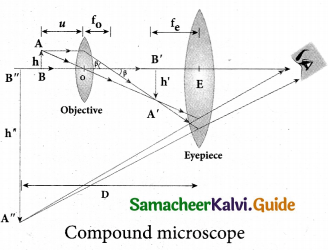Magnification of compound microscope
From the ray diagram, the linear magnification due to the objective is,
$$m_{0}=\frac{h^{\prime}}{h}$$
from the figure ,$$\tan \beta=\frac{h}{f_{0}}=\frac{h^{\prime}}{L}$$, then
$$\frac{h^{\prime}}{h}=\frac{L}{f_{0}} ; m_{o}=\frac{L}{f_{o}}$$

Here, the distance L is between the first focal point of the eyepiece to the second focal point of the objective. This is called the tube length L of the microscope as fo and fe are comparatively smaller than L. If the final image is formed at P (near point focusing), the magnification me of the eyepiece is,
$$m_{e}=1+\frac{D}{f_{e}}$$

The total magnification m in near point focusing is,
$$m=m_{o} m_{e}=\left(\frac{L}{f_{o}}\right)\left(1+\frac{D}{f_{e}}\right)$$

If the final image is formed at infinity (normal focusing), the magnification me of the eyepiece is
$$m_{e}=\frac{D}{f_{e}}$$

The total magnification m in normal focusing is,
$$m=m_{o} m_{e}=\left(\frac{L}{f_{o}}\right)\left(\frac{D}{f_{e}}\right)$$

Question 37.
(a) Briefly explain the principle and working of electron microscope.
Electron Microscope:
Principle:

• This is the direct application of wave nature of particles. The wave nature of the electron is used in the construction of microscope called electron microscope.
• The resolving power of a microscope is inversely proportional to the wavelength of the radiation used for illuminating the object under study. Higher magnification as well as higher resolving power can be obtained by employing the waves of shorter wavelengths.
• De Broglie wavelength of electron is very much less than (a few thousands less) that of the visible light being used in optical microscopes.
• As a result, the microscopes employing de Broglie waves of electrons have very much higher resolving power than optical microscope.
• Electron microscopes giving magnification more than 2,00,000 times are common in research laboratories.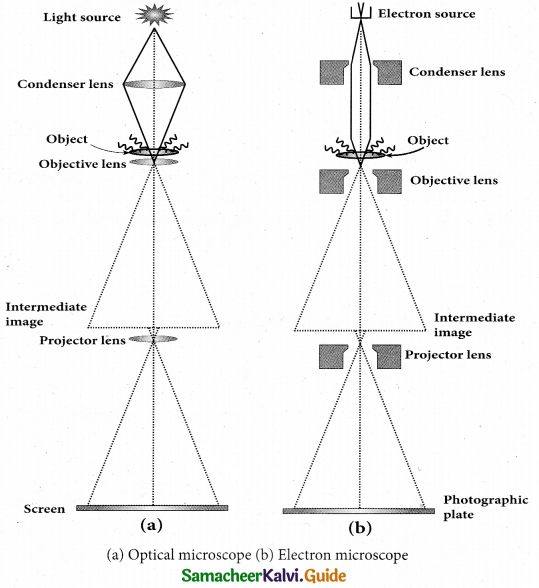Working:

• The electron beam passing across a suitably arranged either electric or magnetic fields undergoes divergence or convergence thereby focussing of the beam is done.
• The electrons emitted from the source are accelerated by high potentials. The beam is made parallel by magnetic condenser lens. When the beam passes through the sample whose magnified image is needed, the beam carries the image of the sample.
• With the help of magnetic objective lens and magnetic projector lens system, the magnified image is obtained on the screen. These electron microscopes are being used in almost all branches of science.

[OR]

(b) Discuss the process of nuclear fission and its properties.

• When uranium nucleus is bombarded with a neutron, it breaks up into two smaller nuclei of comparable masses with the release of energy.
• The process of breaking up of the nucleus of a heavier atom into two smaller nuclei with the release of a large amount of energy is called nuclear fission.
• The fission is accompanied by the release of neutrons. The energy that is released in the nuclear fission is of many orders of magnitude greater than the energy released in chemical reactions.
• Uranium undergoes fission reaction in 90 different ways. The most common fission reactions of• Here Q is energy released during the decay of each uranium nuclei. When the slow neutron is absorbed by the uranium nuclei, the mass number increases by one and goes to an excited state. $$_{ 92 }^{ 236 }{ U }$$ . But this excited state does not last longer than 10-12s and decay into two daughter nuclei along with 2 or 3 neutrons. From each reaction, on an average, 2.5 neutrons are emitted.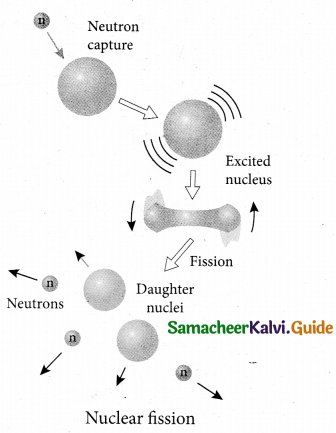Question 38.
(a) Transistor functions as a switch. Explain.
The transistor in saturation and cut-off regions functions like an electronic switch that helps to. turn ON or OFF a given circuit by a small control signal.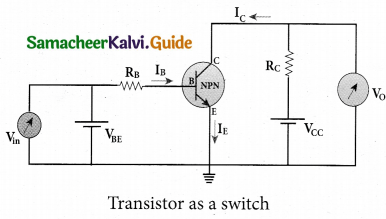Presence of dc source at the input (saturation region):
When a high input voltage (V. = +5V) is applied, the base current (I ) increases and in turn increases the collector current. The transistor will move into the saturation region (turned ON). The increase in collector current (Ic) increases the voltage drop across Rc .thereby lowering the output voltage, close to zero. The transistor acts like a closed switch and is equivalent to ON condition.

Absence of dc source at the input (cut-off region):
A low input voltage (Vin = OV), decreases the base current (IB) and in turn decreases the collector current (Ic ). The transistor will move into the cut-off region (turned OFF). The decrease in collector current (Ic) decreases the drop across, thereby increasing the output voltage, dose to +5 V. The transistor acts as an open switch which is considered as the OFF condition.

It is manifested that, a high input gives a low output and a low input gives a high output. In addition, we can say that the output voltage is opposite to the applied input voltage. Therefore, a transistor can be used as an inverter in computer logic circuitry[OR]

(b) What is modulation? Explain the types of modulation with necessary diagrams.
Modulation: For long distance transmission, the low frequency baseband signal (input signal) is superimposed onto a high frequency radio signal by a process called modulation. There are 3 types of modulation based on which parameter is modified.
They are

1. Amplitude modulation,
2. Frequency modulation, and
3. Phase modulation.

1. Amplitude Modulation (AM): If the amplitude of the carrier signal is modified according to the instantaneous amplitude of the baseband signal, then it is called amplitude modulation. Here the frequency and the phase of the carrier signal remain constant. Amplitude modulation is used in radio and TV broadcasting.

The signal shown in figure
(a) is the message signal or baseband signal that carries information, figure
(b) shows the high-frequency carrier signal and figure
(c) gives the amplitude modulated signal. We can see clearly that the carrier wave is modified in proportion to the amplitude of the baseband signal.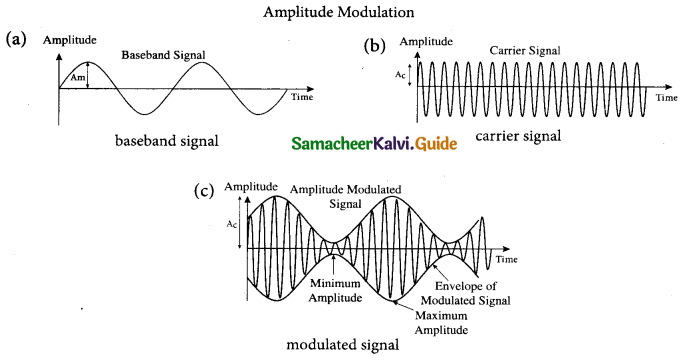(ii) Frequency Modulation (FM):
The frequency of the carrier signal is modified according to the instantaneous amplitude of the baseband signal in frequency modulation. Here the amplitude and the phase of the carrier signal remain constant. An increase in the amplitude of the ‘ baseband signal increases the frequency of the carrier signal and vice versa. This leads to compressions and rarefactions in the frequency spectrum of the modulated wave.Louder signal leads to compressions and relatively weaker signals to rarefactions. When the amplitude of the baseband signal is zero in Figure (a), the frequency of the modulated signal is the same as the carrier signal. The frequency of the modulated wave increases when the amplitude of the baseband signal increases in the positive direction (A, C). The increase in amplitude in the negative half cycle (B, D) reduces the frequency of the modulated wave (Figure (c)).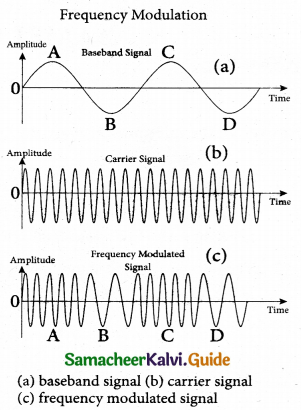(iii) Phase Modulation (PM)
The instantaneous amplitude of the baseband signal modifies the phase of the carrier signal keeping the amplitude and frequency constant is called phase modulation. This modulation is used to generate frequency modulated signals. It is similar to frequency modulation except that the phase of the carrier is varied instead of varying frequency.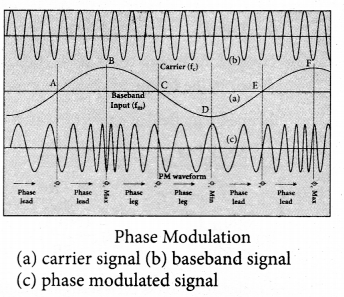The carrier phase changes according to increase or decrease in the amplitude of the baseband signal. When the modulating signal goes positive, the amount of phase lead increases with the amplitude of the modulating signal. Due to this, the carrier signal is compressed or its frequency is increased.

On the other hand, the negative half cycle of the baseband signal produces a phase lag in the carrier signal. This appears to have stretched the frequency of the carrier wave. Hence similar to frequency modulated wave, phase modulated wave also comprises of compressions and rarefactions. When the signal voltage is zero (A, C and E) the carrier frequency is unchanged.